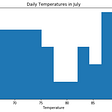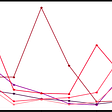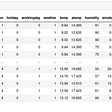# Vector Auto-Regressive (VAR) Models for Multivariate Time Series Forecasting

The Vector Auto Regression (VAR) model is one of the most successful, flexible, and easy to use models for the analysis of multivariate time series. It is a natural extension of the univariate autoregressive model to dynamic multivariate time series. The VAR model has proven to be especially useful for describing the dynamic behavior of economic and financial time series and for forecasting. It often provides superior forecasts to those from univariate time series models and elaborate theory-based simultaneous equations models. Forecasts from VAR models are quite flexible because they can be made conditional on the potential future paths of specified variables in the model.

In addition to data description and forecasting, the VAR model is also used for structural inference and policy analysis. In structural analysis, certain assumptions about the causal structure of the data under investigation are imposed, and the resulting causal impacts of unexpected shocks or innovations to specified variables on the variables in the model are summarized. These causal impacts are usually summarized with impulse response functions and forecast error variance decompositions.

This article assumes that we have knowledge of basic time series models like ARMA, ARIMA, SARIMAX, etc.

# Introduction

Vector Autoregression (VAR) is a forecasting algorithm that can be used when two or more time series influence each other, i.e. the relationship between the time series involved is bi-directional. It is considered as an Autoregressive model because, each variable (Time Series) is modeled as a function of the past values, that is the predictors are nothing but the lags (time delayed value) of the series.

Other Autoregressive models like AR, ARMA or ARIMA are uni-directional, where, the predictors influence the Y and not vice-versa. Vector Auto Regression (VAR) models are bi-directional, i.e. the variables influence each other.

# Types of VAR Models

There are three broad types of VAR models, the reduced form, the recursive form, and the structural VAR model.

1. Reduced form VAR models: These models consider each variable to be a function of it’s own past values, the past values of all other variables being considered and a serially uncorrelated error term. The error terms will be correlated across equations in these models. This means we cannot consider what impacts individual shocks will have on the system.
2. Recursive VAR models: These models contain all the components of the reduced form model, but also allow some variables to be functions of other concurrent variables. By imposing these short-run relationships, the recursive model allows us to model structural shocks. A recursive VAR constructs the error terms in each regression equation to be
uncorrelated with the error in the preceding equations. This is done by judiciously including some contemporaneous values as regressors.
3. Structural VAR models: These models include restrictions that allow us to identify causal relationships beyond those that can be identified with reduced form or recursive models. These causal relationships can be used to model and forecast impacts of individual shocks, such as policy decisions.

# Defining the Model

Basically, a VAR model implies that everything depends on everything. VAR model can be defined as:

Assumptions:

• yt: Stationary K-variable vector
• v: K constant parameters vector
• Aj: K by K parameters matrix, j=1,….,p
• ut: i.i.d.(0,Sigma)
• Trend may be included: delta(t), where delta is K by 1
• Exogenous variables X may be added

The equation can be estimated using ordinary least squares given a few assumptions:

• The error term has a conditional mean of zero.
• The variables in the model are stationary.
• Large outliers are unlikely.
• No perfect multicollinearity.

Under these assumptions, the ordinary least squares estimates:

• Will be consistent.
• Can be evaluated using traditional t-statistics and p-values.
• Can be used to jointly test restrictions across multiple equations.

A VAR model is made up of a system of equations that represents the relationships between multiple variables. When referring to VAR models, we often use special language to specify:

• Number of endogenous variables.
• Number of autoregressive terms.

For example, if we have two endogenous variables and autoregressive terms, we say the model is a Bivariate VAR(2) model. If we have three endogenous variables and four autoregressive terms, we say the model is a Trivariate VAR(4) model. In general, a VAR model is composed of n-equations (representing n endogenous variables) and includes p-lags of the variables.

# Lag Selection

Lag selection is one of the important aspects of VAR model specification. In practical applications, we generally choose a maximum number of lags, p(max), and evaluate the performance of the model including p = 0,1,….,p(max). The optimal model is then the model VAR(p) which minimizes some lag selection criteria.

where:

• Yt = (y1t,y2t,…..,ynt) is an (n * 1) vector of time series variables
• a = an (n * 1) vector of intercepts
• Ai (i=1,2,…,p) = (n * n) coefficient matrices
• Ei = an (n * 1) vector of unobservable i.i.d. zero mean error term (white noise)

The most commonly used lag selection criteria are:

• Akaike (AIC)
• Schwarz-Bayesian (BIC)
• Hannan-Quinn (HQ)
• Final Prediction Error (FPE)
• Average of the above

These methods are usually built into libraries and lag selection is almost completely automated now.

# Variable Selection, Forecasting and Evaluation

The forecasting relevance of endogenous variables can be tested using Granger-causality test, Wald Test, etc. If you have more than two variables you should consider multivariate Granger-causality style testing using a VAR. You should also consider whether variables are stationary or nonstationary. If variables are stationary you can apply Granger-causality testing in a stationary VAR. If the variables are nonstationary and not cointegrated you can difference the variables and apply Granger-causality testing in a stationary VAR of the differenced variables . If the variables are nonstationary and are cointegrated you can apply Granger-causality testing in a VECM (that has short-run and long-run components) that assumes all the data are stationary by either cointegration or differencing transformations. If there is no cointegration the error-correction term can be excluded and testing is conducted by a Wald test in a stationary VAR. Alternatively, you can use the Toda and Yamamoto (1995) surplus lag Granger-causality test that uses nonstationary data.

One of the most important functions of VAR models is to generate forecasts. Forecasts are generated for VAR models using an iterative forecasting algorithm:

• Estimate the VAR model using OLS for each equation.
• Compute the one-period-ahead forecast for all variables.
• Iterate until the h-step ahead forecasts are computed.

Often we are more interested in the dynamics that are predicted by our VAR models than the actual coefficients that are estimated. For this reason, it is most common that VAR studies report:

• Granger-causality statistics.
• Impulse response functions.
• Forecast error decompositions

# Implementation in SAS

Let us take an example DJIA index and total market capitalization to see how VAR works in SAS.

• Testable hypothesis: there has to be a dependence of DJIA index on its own lag and on lag of total market capitalization and vice versa.
• Use return on DJIA index and return on market capitalization
• Monthly observation

The dataset and the implementation is given below:

For implementation in python refer to the official documentation of statsmodels.tsa.vector_ar.

# Criticisms and Usefulness

A criticism that VARs face is that they are atheoretical; that is, they are not built on some economic theory that imposes a theoretical structure on the equations. Every variable is assumed to influence every other variable in the system, which makes a direct interpretation of the estimated coefficients difficult. Despite this, VAR models are useful in several contexts:

• forecasting a collection of related variables where no explicit interpretation is required;
• testing whether one variable is useful in forecasting another (the basis of Granger causality tests);
• impulse response analysis, where the response of one variable to a sudden but temporary change in another variable is analyzed;
• forecast error variance decomposition, where the proportion of the forecast variance of each variable is attributed to the effects of the other variables.

I hope this article gave you a perspective on this advanced and useful technique for dealing with multi-variate time series data. I am looking forward to your valuable feedback/comments, as it helps me to plan and research my articles better. Please feel free to comment any topic in Machine Learning that you would want me to write an article on.

Reference:
www.aptech.com

--

--

--

## More from Geek Culture

A new tech publication by Start it up (https://medium.com/swlh).

## Before you start a data science project ask your users this one question## Prediction of P-Sonic Log in the Volve Oil Field using Machine Learning## Data Analytics — A brief Introduction## Six Recommendations for Aspiring Data Scientists## Isn’t DataOps Is Just DevOps for Data?## A Graph on COVID-19 cases in Singapore## Into the data: Day 7## A Brief Introduction to Plotting in Python## Aman Gupta

Is a pantomath and a former entrepreneur. Currently, he is in a harmonious and a symbiotic relationship with Data.

## Time-Series Analysis on Practical dataset: Don’t jump into fitting models!## Time Series Forecasting with Supervised Machine Learning## Udemy- Python for Time Series Data Analysis Course Review## Multivariate Time Series Forecasting using Vector AutoRegression